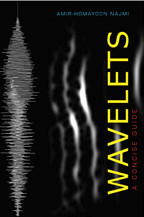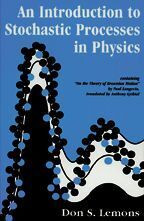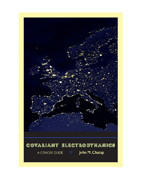Back to Results# Wavelets

A Concise Guide

Amir-Homayoon Najmi

Publication Date
Request Exam CopyRequest Review Copy

Introduced nearly three decades ago as a variable resolution alternative to the Fourier transform, a wavelet is a short oscillatory waveform for analysis of transients. The discrete wavelet transform has remarkable multi-resolution and energy-compaction properties. Amir-Homayoon Najmi’s introduction to wavelet theory explains this mathematical concept clearly and succinctly.

Wavelets are used in processing digital signals and imagery from myriad sources. They form the backbone of the JPEG2000 compression standard, and the Federal Bureau of Investigation uses biorthogonal wavelets to compress…

Introduced nearly three decades ago as a variable resolution alternative to the Fourier transform, a wavelet is a short oscillatory waveform for analysis of transients. The discrete wavelet transform has remarkable multi-resolution and energy-compaction properties. Amir-Homayoon Najmi’s introduction to wavelet theory explains this mathematical concept clearly and succinctly.

Wavelets are used in processing digital signals and imagery from myriad sources. They form the backbone of the JPEG2000 compression standard, and the Federal Bureau of Investigation uses biorthogonal wavelets to compress and store its vast database of fingerprints. Najmi provides the mathematics that demonstrate how wavelets work, describes how to construct them, and discusses their importance as a tool to investigate and process signals and imagery. He reviews key concepts such as frames, localizing transforms, orthogonal and biorthogonal bases, and multi-resolution. His examples include the Haar, the Shannon, and the Daubechies families of orthogonal and biorthogonal wavelets.

Our capacity and need for collecting and transmitting digital data is increasing at an astonishing rate. So too is the importance of wavelets to anyone working with and analyzing digital data. Najmi’s primer will be an indispensable resource for those in computer science, the physical sciences, applied mathematics, and engineering who wish to obtain an in-depth understanding and working knowledge of this fascinating and evolving field.

Wavelets

Amir-Homayoon Najmi

Publication Date
Request Exam CopyRequest Review Copy
Related

## Related BooksAn Introduction to Stochastic Processes in Physics

Don S. Lemons
containing "On the Theory of Brownian Motion" by Paul Langevin, translated by Anthony Gythiel

\$34.00
An Introduction to Stochastic Processes in Physics

Don S. Lemons
containing "On the Theory of Brownian Motion" by Paul Langevin, translated by Anthony Gythiel

Publication DateCovariant Electrodynamics

John M. Charap

\$35.00
Covariant Electrodynamics

John M. Charap

Publication DateQuantum Steampunk

Nicole Yunger Halpern

\$29.95
Quantum Steampunk

Nicole Yunger Halpern

Publication DateRenewable Energy

Stephen Peake

\$14.95
Renewable Energy

Stephen Peake

Publication DateTime Travel

Brian Clegg

\$14.95
Time Travel

Brian Clegg

Publication Date
Reviews

## Reviews

A complete, concise and clear exposition of the more traditional tools related to linear filtering.

Since their emergence in the last eighties and early nineties of the twentieth century, wavelets and other multi-scale transforms have become powerful signal and image processing tools. Najmi's book provides physicists and engineers with a clear and concise introduction to this fascinating field.

## Book Details

Publication Date
Status
Available
Trim Size
6.125
x
9.25
Pages
304
ISBN
9781421404967
Illustration Description
9 halftones, 88 line drawings
Subject

List of Tables
List of Figures
List of Acronyms
Preface
Acknowledgments
1. Analysis in Vector and Function Spaces
1.1. Introduction
1.2. The Lebesgue Integral
1.3. Discrete Time Signals
1.4. Vector Spaces
1.5

List of Tables
List of Figures
List of Acronyms
Preface
Acknowledgments
1. Analysis in Vector and Function Spaces
1.1. Introduction
1.2. The Lebesgue Integral
1.3. Discrete Time Signals
1.4. Vector Spaces
1.5. Linear Independence
1.6. Bases and Basis Vectors
1.7. Normed Vector Spaces
1.8. Inner Product
1.9. Banach and Hilbert Spaces
1.10. Linear Operators, Operator Norm, the Adjoint Operator
1.11. Reproducing Kernel Hilbert Space
1.12. The Dirac Delta Distribution
1.13. Orthonormal Vectors
1.14. Orthogonal Projections
1.15. Multi-Resolution Analysis Subspaces
1.16. Complete and Orthonormal Bases in L2 (R)
1.17. The Dirac Notation
1.18. The Fourier Transform
1.19. The Fourier Series Expansion
1.20. The Discrete Time Fourier Transform
1.21. The Discrete Fourier Transform
1.22. Band-Limited Functions and the Sampling Theorem
1.23. The Basis Operator in L2(R)
1.24. Biorthogonal Bases and Representations in L2 (R)
1.25. Frames in a Finite Dimensional Vector Space
1.26. Frames in L2 (R)
1.27. Dual Frame Construction Algorithm
1.28. Exercises
2. Linear Time-Invariant Systems
2.1. Introduction
2.2. Convolution in Continuous Time
2.3. Convolution in Discrete Time
2.4. Convolution of Finite Length Sequences
2.5. Linear Time-Invariant Systems and the Z Transform
2.6. Spectral Factorization for Finite Length Sequences
2.7. Perfect Reconstruction Quadrature Mirror Filters
2.8. Exercises
3. Time, Frequency, and Scale Localizing Transforms
3.1. Introduction
3.2. The Windowed Fourier Transform
3.3. The Windowed Fourier Transform Inverse
3.4. The Range Space of the Windowed Fourier Transform
3.5. The Discretized Windowed Fourier Transform
3.6. Time-Frequency Resolution of theWindowed Fourier Transform
3.7. The Continuous Wavelet Transform
3.8. The Continuous Wavelet Transform Inverse
3.9. The Range Space of the Continuous Wavelet Transform
3.10. The Morlet, the Mexican Hat, and the Haar Wavelets
3.11. Discretizing the Continuous Wavelet Transform
3.12. Algorithm A' Trous
3.13. The Morlet Scalogram
3.14. Exercises
4. The Haar and Shannon Wavelets
4.1. Introduction
4.2. Haar Multi-Resolution Analysis Subspaces
4.3. Summary and Generalization of Results
4.4. The Spectra of the Haar Filter Coefficients
4.5. Half-Band Finite Impulse Response Filters
4.6. The Shannon Scaling Function
4.7. The Spectrum of the Shannon Filter Coefficients
4.8. Meyer's Wavelet
4.9. Exercises
5. General Properties of Scaling and Wavelet Functions
5.1. Introduction
5.2. Multi-Resolution Analysis Spaces
5.3. The Inverse Relations
5.4. The Shift-Invariant Discrete Wavelet Transform
5.5. Time Domain Properties
5.6. Examples of Finite Length Filter Coefficients
5.7. Frequency Domain Relations
5.8. Orthogonalization of a Basis Set: b1 Spline Wavelet
5.9. The Cascade Algorithm
5.10. Biorthogonal Wavelets
5.11. Multi-Resolution Analysis Using Biorthogonal Wavelets
5.12. Exercises
6. Discrete Wavelet Transform of Discrete Time Signals
6.1. Introduction
6.2. Discrete Time Data and Scaling Function Expansions
6.3. Implementing the DWT for Even Length h0 Filters
6.4. Denoising and Thresholding
6.5. Biorthogonal Wavelets of Compact Support
6.6. The Lazy Filters
6.7. Exercises
7. Wavelet Regularity and Daubechies Solutions
7.1. Introduction
7.2. Zero Moments of the Mother Wavelet
7.3. The Form of H0(z) and the Decay Rate of F(?)
7.4. Daubechies Orthogonal Wavelets of Compact Support
7.5. Wavelet and Scaling Function Vanishing Moments
7.6. Biorthogonal Wavelets of Compact Support
7.7. Biorthogonal Spline Wavelets
7.8. The Lifting Scheme
7.9. Exercises
8. Orthogonal Wavelet Packets
8.1. Introduction
8.2. Review of the Orthogonal Wavelet Transform
8.3. Packet Functions for Orthonormal Wavelets
8.4. Discrete Orthogonal Packet Transform of Finite Length Sequences
8.5. The Best Basis Algorithm
8.6. Exercises
9. Wavelet Transform in Two Dimensions
9.1. Introduction
9.2. The Forward Transform
9.3. The Inverse Transform
9.4. Implementing the Two-Dimensional Wavelet Transform
9.5. Application to Image Compression
9.6. Image Fusion
9.8. Exercises
Bibliography
Index

Author Bio
Featured Contributor

## Amir-Homayoon Najmi

Amir-Homayoon Najmi completed the Mathematical Tripos at Cambridge University and obtained his D.Phil. at Oxford University. He is with the Johns Hopkins University's Applied Physics Laboratory and is a faculty member of the Whiting School of Engineering CE programs in applied physics and electrical engineering.
Resources